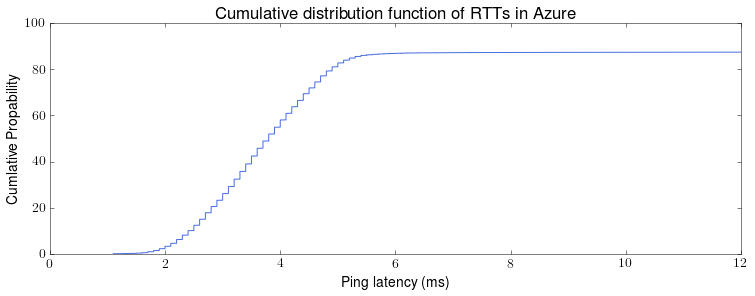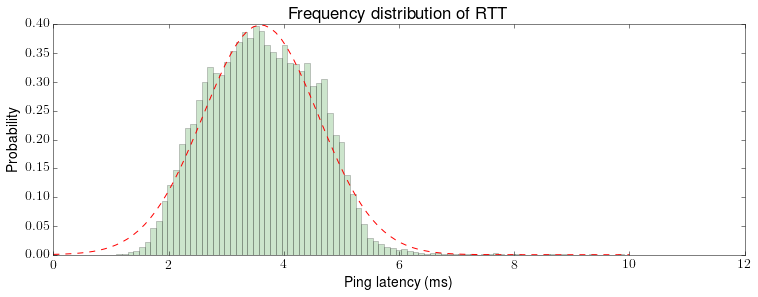# Azure Latency Pilot Study: Part 2 – Aggregated results

This is post we will be looking at the results for the Azure latency Pilot study described last week. We will starting by looking at the aggregate results, disregarding the time a measurement was taken and which machines the measurement was taken between.

The 22332 data points have been processed in Python3, in particular using the matplotlib and numpy libraries. The scripts are available in azure-measurements repository.
They currently only use the average round trip time, as reported by hping3, average over 10 pings.

property RTT (ms)
min 1.1
max 1242.2
mean 15.9
std 66.5

The results in the table above are much larger than I expected. Given the large standard deviation and very large maximum value, it is likely that a few large measures have skewed the results. Let’s take a look at the cumulative distribution function (CDF) and percentile points to see if this is the case.

percentile RTT (ms)
25th 3.0
50th 3.8
75th 4.7
90th 61.4
95th 69.7
99th 87.3As expect, some large measurements have skewed the results. However, the proportion of measurement which are considered large is much greater then I expected. This warrants further investigation.

Before that, lets take a closer look at how the majority of value are distributed. Due to the central limit theorem and the sufficiently large sample size, we would expect to see a normal distribution. In simulators such as Raft Refloated, we simulate latency as normally distributed with given parameters, discarding values below a threshold value. We can take a closer look at the probability density function (PDF) and see if this is a reasonable approximation.The green bars represent the probability of each RTT. We see an approximate normal distribution, although it is clear that this distribution doesn’t have the same parameters as the data set as a whole. The red lines shows a normal distributed with mean 3.6 and standard deviation of 1. This red line appears to be a reasonable approximation and could be used in simulation.

Next post, we will looks at how the measured RTT differs depending which of the 5 machines the measurement was taken between.